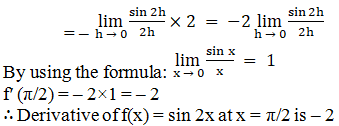# RD Sharma Solutions for Class 11 Chapter 30 - Derivatives Exercise 30.1

Here, in this exercise, we shall discuss concepts related to derivative at a point where the answers are explained with suitable examples for students to understand clearly. Students can make use of RD Sharma Class 11 Maths Solutions to obtain good marks in the board examination. Subject experts have designed the solutions in a step by step manner to help students understand the concepts and score well in the exams. The pdf of RD Sharma Solutions are provided in the links given below, which can be downloaded easily and can be used for future reference as well.

## Download the pdf of RD Sharma Solutions for Class 11 Maths Exercise 30.1 Chapter 30 – Derivatives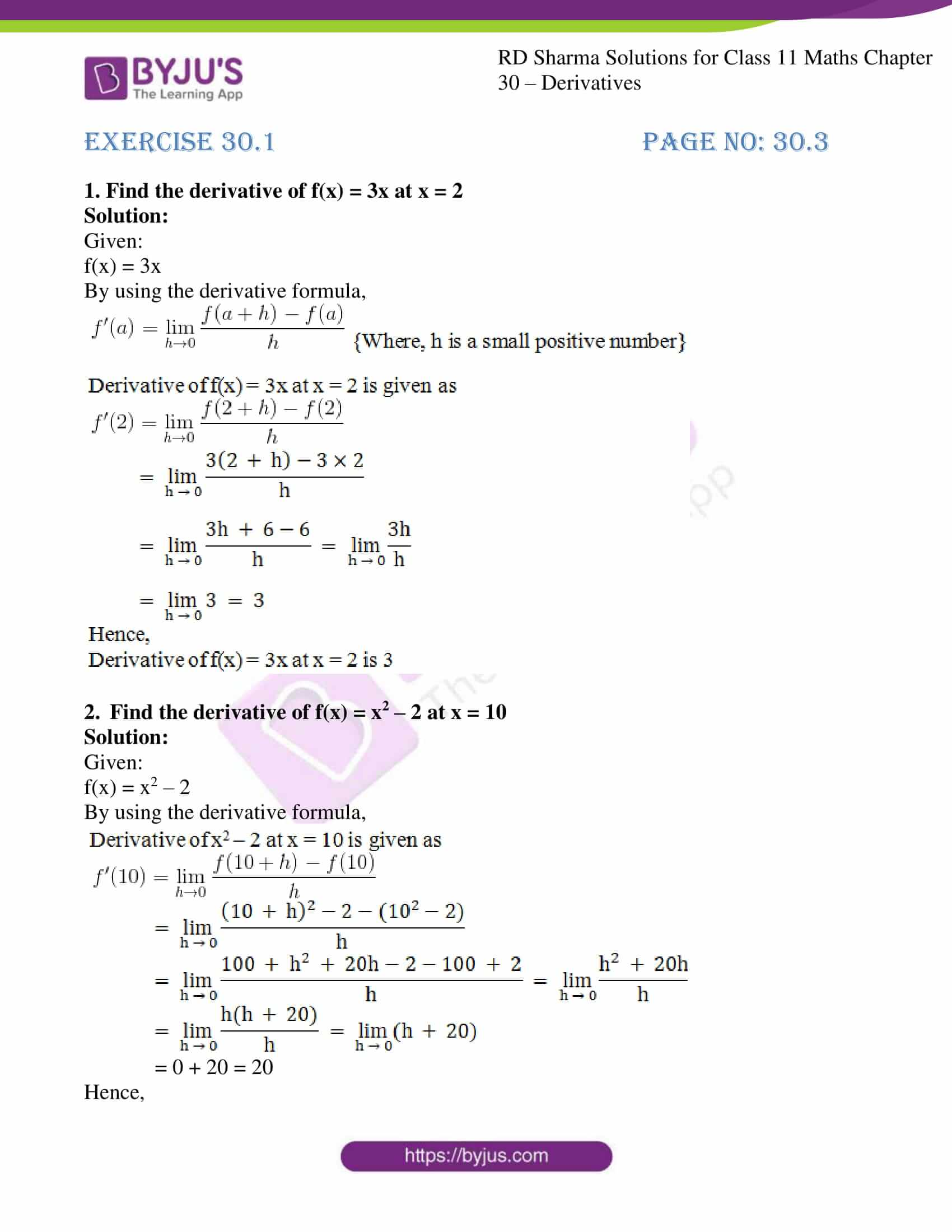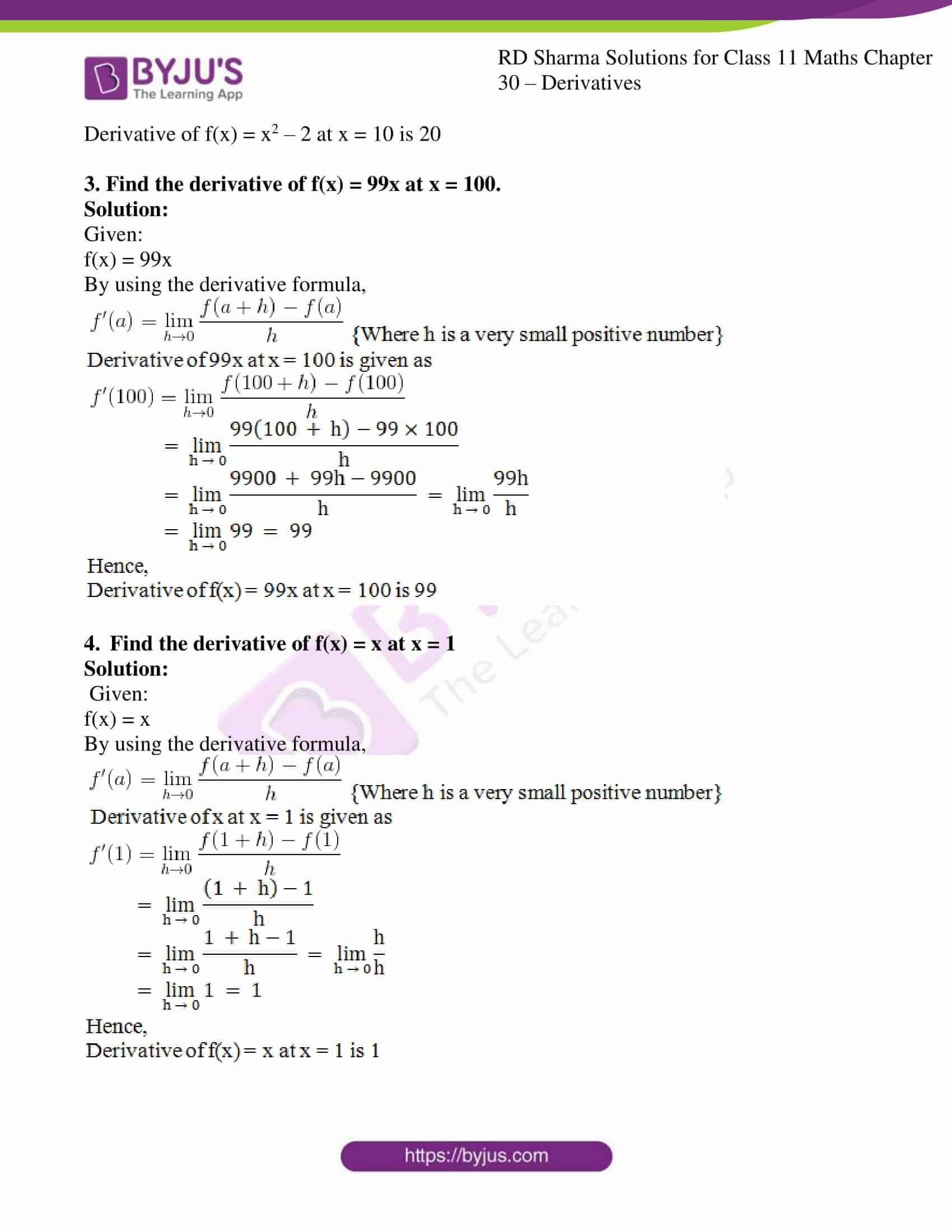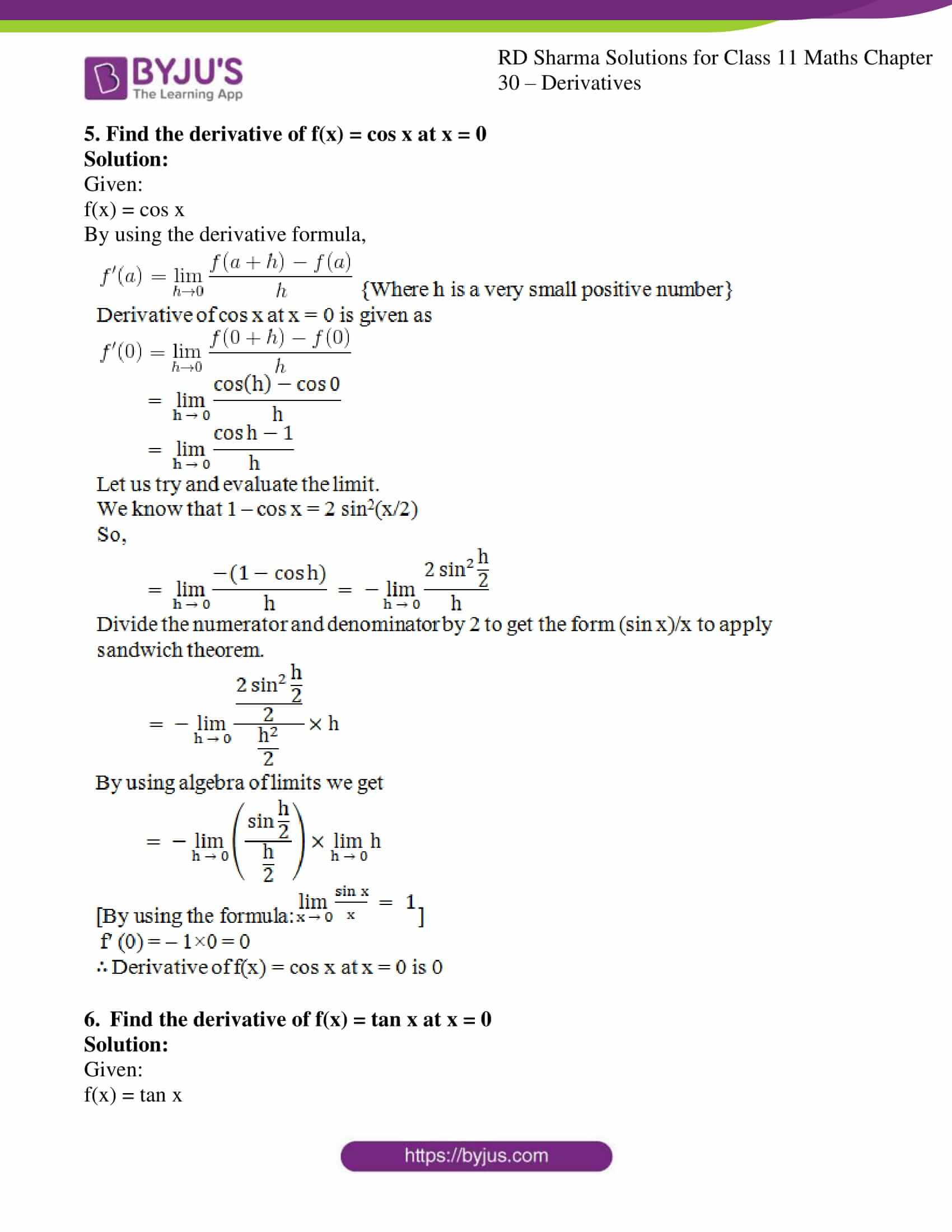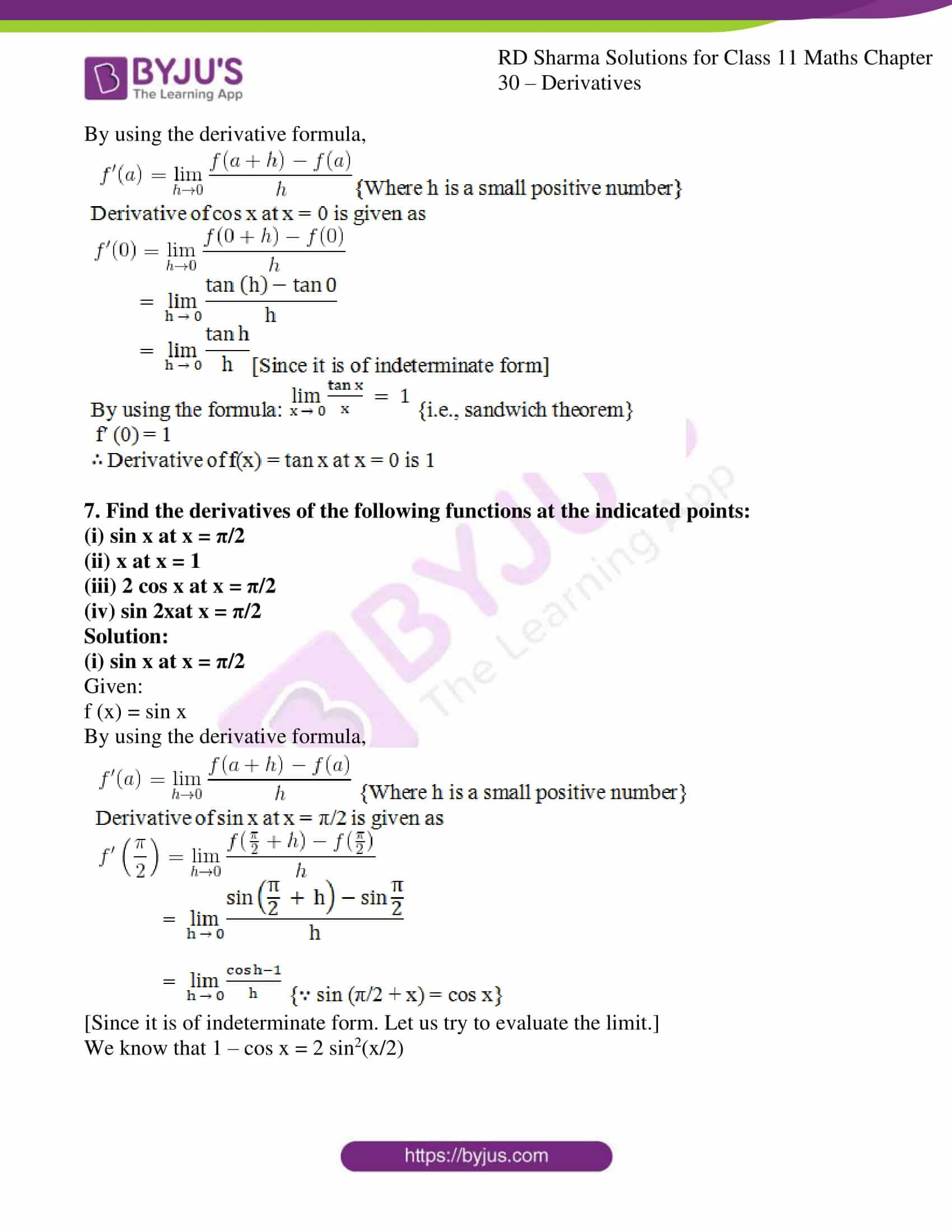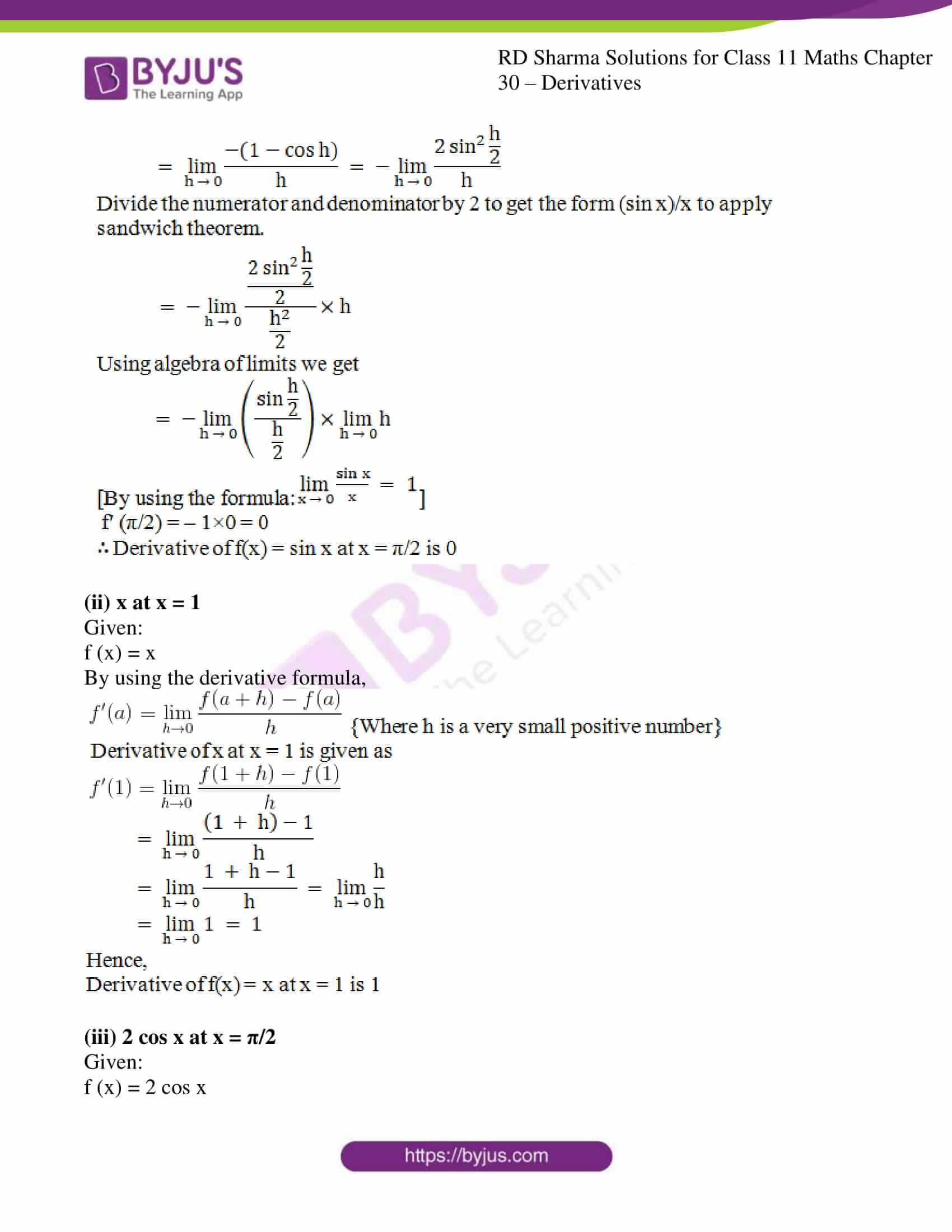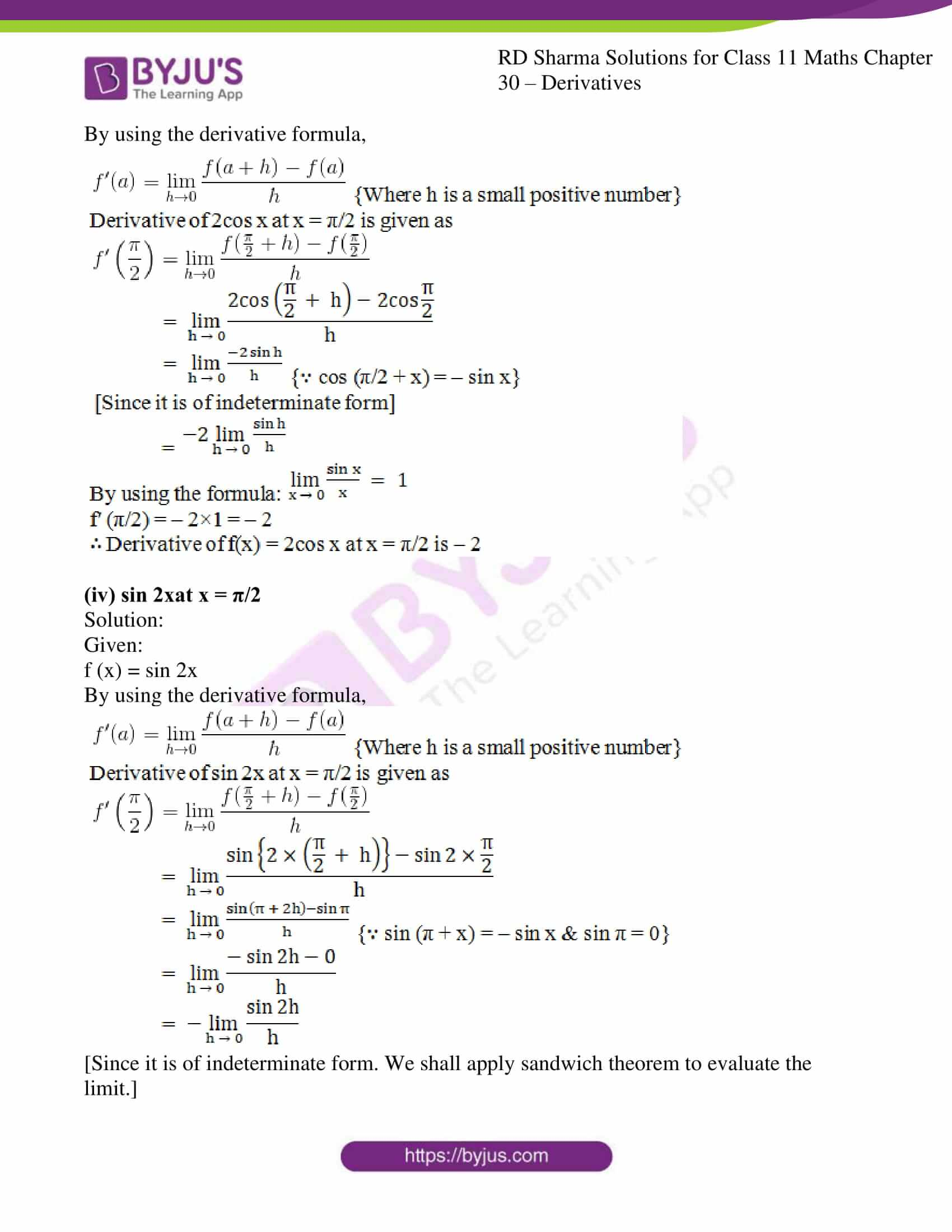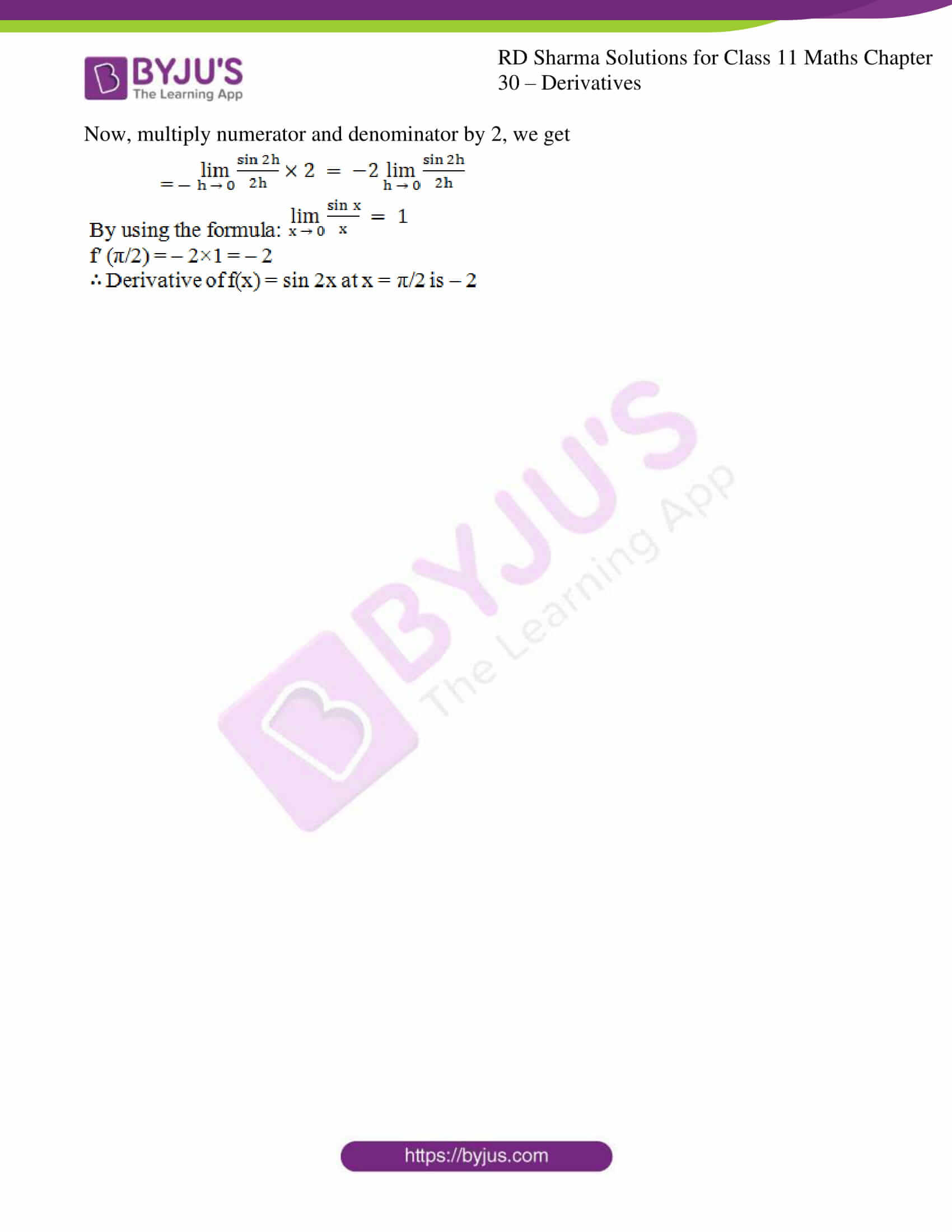### Access answers to RD Sharma Solutions for Class 11 Maths Exercise 30.1 Chapter 30 – Derivatives

#### EXERCISE 30.1 PAGE NO: 30.3

1. Find the derivative of f(x) = 3x at x = 2

Solution:

Given:

f(x) = 3x

By using the derivative formula,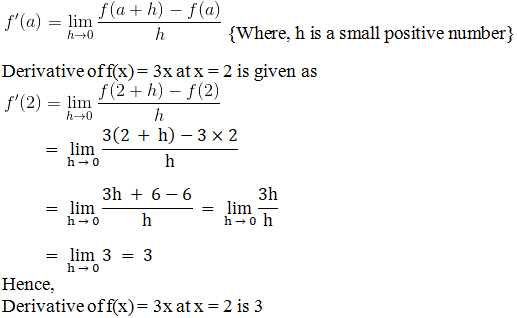2. Find the derivative of f(x) = x2 – 2 at x = 10

Solution:

Given:

f(x) = x2 – 2

By using the derivative formula,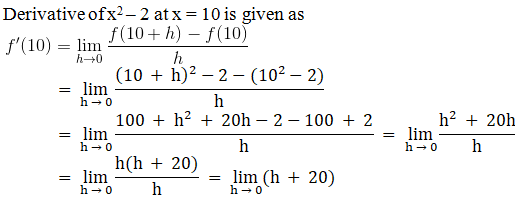= 0 + 20 = 20

Hence,

Derivative of f(x) = x2 – 2 at x = 10 is 20

3. Find the derivative of f(x) = 99x at x = 100.

Solution:

Given:

f(x) = 99x

By using the derivative formula,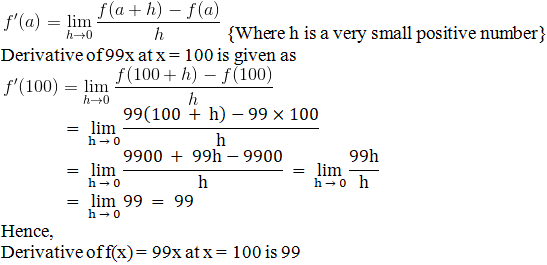4. Find the derivative of f(x) = x at x = 1

Solution:

Given:

f(x) = x

By using the derivative formula,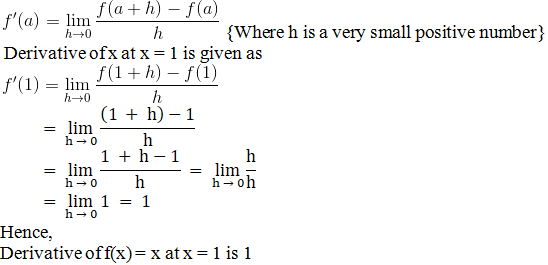5. Find the derivative of f(x) = cos x at x = 0

Solution:

Given:

f(x) = cos x

By using the derivative formula,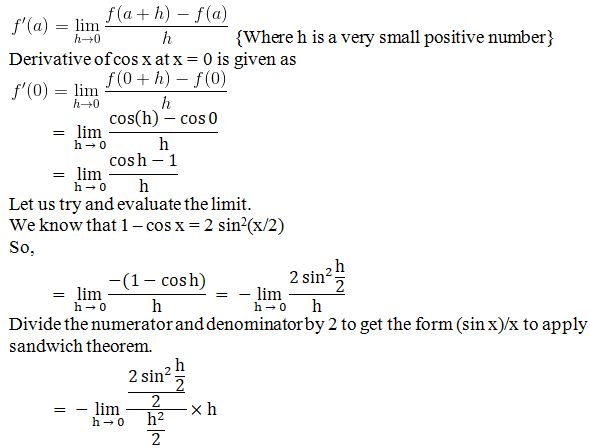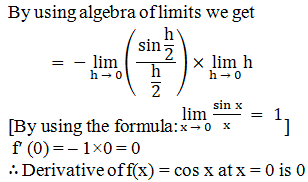6. Find the derivative of f(x) = tan x at x = 0

Solution:

Given:

f(x) = tan x

By using the derivative formula,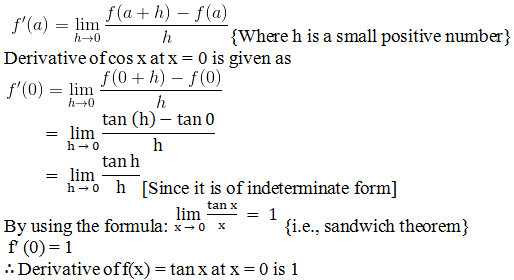7. Find the derivatives of the following functions at the indicated points:
(i) sin x at x = π/2
(ii) x at x = 1

(iii) 2 cos x at x = π/2

(iv) sin 2xat x = π/2

Solution:

(i) sin x at x = π/2

Given:

f (x) = sin x

By using the derivative formula,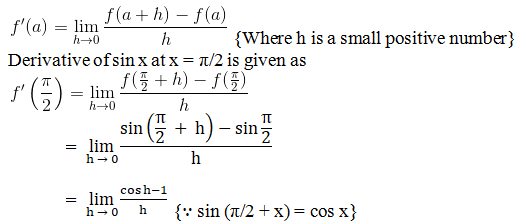[Since it is of indeterminate form. Let us try to evaluate the limit.]

We know that 1 – cos x = 2 sin2(x/2)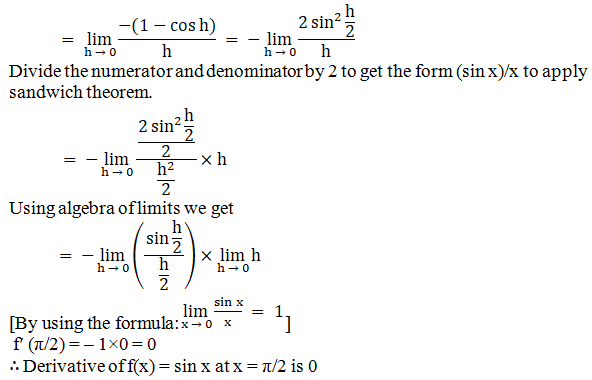(ii) x at x = 1

Given:

f (x) = x

By using the derivative formula,(iii) 2 cos x at x = π/2

Given:

f (x) = 2 cos x

By using the derivative formula,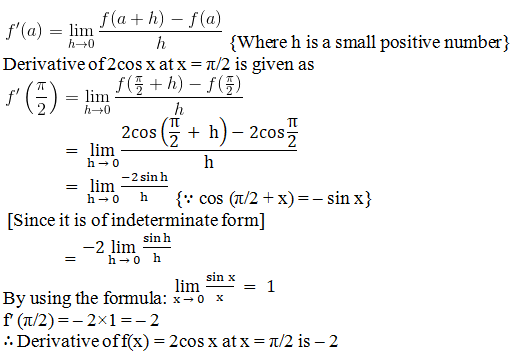(iv) sin 2xat x = π/2

Solution:

Given:

f (x) = sin 2x

By using the derivative formula,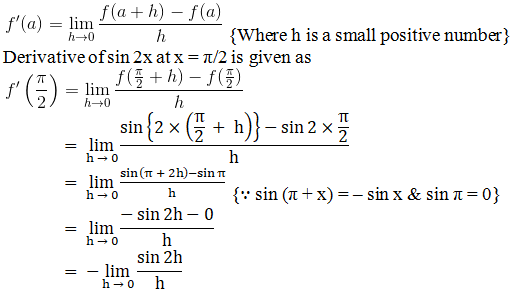[Since it is of indeterminate form. We shall apply sandwich theorem to evaluate the limit.]

Now, multiply numerator and denominator by 2, we get